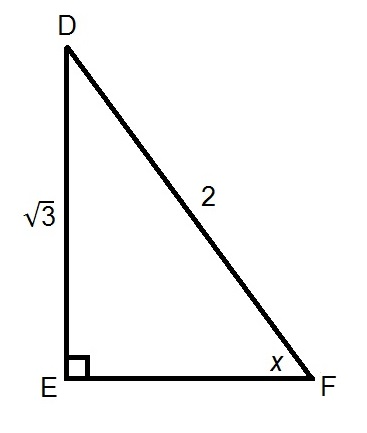# A Geometry Problem

Geometry Level 2In triangle $DEF$, $DE = \sqrt{3}$ and $DF = 2$. What is the value of $\tan x$?

×